Home

# VALUE PATTERNS OF MULTIPLICATIVE FUNCTIONS AND RELATED SEQUENCES

## Abstract

We study the existence of various sign and value patterns in sequences defined by multiplicative functions or related objects. For any set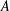$A$ whose indicator function is ‘approximately multiplicative’ and uniformly distributed on short intervals in a suitable sense, we show that the density of the pattern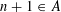$n+1\in A$ ,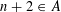$n+2\in A$ ,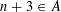$n+3\in A$ is positive as long as$A$ has density greater than$\frac{1}{3}$ . Using an inverse theorem for sumsets and some tools from ergodic theory, we also provide a theorem that deals with the critical case of$A$ having density exactly$\frac{1}{3}$ , below which one would need nontrivial information on the local distribution of$A$ in Bohr sets to proceed. We apply our results first to answer in a stronger form a question of Erdős and Pomerance on the relative orderings of the largest prime factors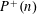$P^{+}(n)$ ,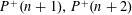$P^{+}(n+1),P^{+}(n+2)$ of three consecutive integers. Second, we show that the tuple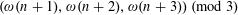$(\unicode[STIX]{x1D714}(n+1),\unicode[STIX]{x1D714}(n+2),\unicode[STIX]{x1D714}(n+3))~(\text{mod}~3)$ takes all the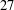$27$ possible patterns in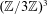$(\mathbb{Z}/3\mathbb{Z})^{3}$ with positive lower density, with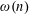$\unicode[STIX]{x1D714}(n)$ being the number of distinct prime divisors. We also prove a theorem concerning longer patterns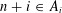$n+i\in A_{i}$ ,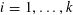$i=1,\ldots ,k$ in approximately multiplicative sets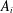$A_{i}$ having large enough densities, generalizing some results of Hildebrand on his ‘stable sets conjecture’. Finally, we consider the sign patterns of the Liouville function$\unicode[STIX]{x1D706}$ and show that there are at least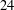$24$ patterns of length$5$ that occur with positive upper density. In all the proofs, we make extensive use of recent ideas concerning correlations of multiplicative functions.

• # Send article to Kindle

Note you can select to send to either the @free.kindle.com or @kindle.com variations. ‘@free.kindle.com’ emails are free but can only be sent to your device when it is connected to wi-fi. ‘@kindle.com’ emails can be delivered even when you are not connected to wi-fi, but note that service fees apply.

Find out more about the Kindle Personal Document Service.

VALUE PATTERNS OF MULTIPLICATIVE FUNCTIONS AND RELATED SEQUENCES
Available formats
×

# Send article to Dropbox

To send this article to your Dropbox account, please select one or more formats and confirm that you agree to abide by our usage policies. If this is the first time you use this feature, you will be asked to authorise Cambridge Core to connect with your <service> account. Find out more about sending content to Dropbox.

VALUE PATTERNS OF MULTIPLICATIVE FUNCTIONS AND RELATED SEQUENCES
Available formats
×

# Send article to Google Drive

To send this article to your Google Drive account, please select one or more formats and confirm that you agree to abide by our usage policies. If this is the first time you use this feature, you will be asked to authorise Cambridge Core to connect with your <service> account. Find out more about sending content to Google Drive.

VALUE PATTERNS OF MULTIPLICATIVE FUNCTIONS AND RELATED SEQUENCES
Available formats
×

This is an Open Access article, distributed under the terms of the Creative Commons Attribution licence (http://creativecommons.org/licenses/by/4.0/), which permits unrestricted re-use, distribution, and reproduction in any medium, provided the original work is properly cited.

## References

Hide All
MathJax
MathJax is a JavaScript display engine for mathematics. For more information see http://www.mathjax.org.

# VALUE PATTERNS OF MULTIPLICATIVE FUNCTIONS AND RELATED SEQUENCES

## Metrics

### Full text viewsFull text views reflects the number of PDF downloads, PDFs sent to Google Drive, Dropbox and Kindle and HTML full text views.

Total number of HTML views: 0
Total number of PDF views: 0 *Loading metrics...

### Abstract viewsAbstract views reflect the number of visits to the article landing page.

Total abstract views: 0 *Loading metrics...

* Views captured on Cambridge Core between <date>. This data will be updated every 24 hours.

Usage data cannot currently be displayed